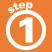Home    |    Teacher    |    Parents    |    Glossary    |    About UsWhat is the ratio of squares to triangles?

We use ratios to make comparisons between two things. When we express ratios in words, we use the word "to" -- we say "the ratio of something to something else" -- for example, the ratio of squares to triangles in the illustration below. Ratios can be written in several different ways.

Click on each word below to see the ratio of squares to triangles expressed in each way.Multiplying or dividing each term by the same nonzero number will give an equal ratio. For example, the ratio 2:4 is equal to the ratio 1:2. To tell if two ratios are equal, use a calculator and divide. If the division gives the same answer for both ratios, then they are equal.

 Homework Help | Pre-Algebra | Ratios and ProportionsEmail this page to a friendSearch·  Ratios·  Proportions·  Distance, rate     and time·  Similar figuresFirst Glance In Depth Examples WorkoutRatios